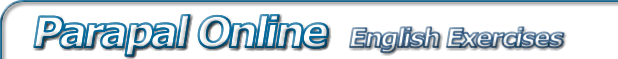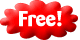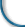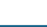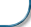part 1 > part 2

# Semi formal business e-mail example. Fill in the gap English exercise. Improve your vocabulary and grammar

Instructions: This informal report has a range of formal and informal vocabulary. It is appropriate for colleagues with a close working relationship.

Hi Doug,

The answers are: 1 = to 2 = with 3 = to 4 = at 5 = with 6 = about 7 = about 8 = about 9 = by 10 = at 11 = to 12 = for 13 = at 14 = to 15 = to 16 = from 17 = about 18 = to 19 = by 20 = to 21 = at 22 = with 23 = at 24 = to 25 = to 26 = for 27 = to 28 = to 29 = to 30 = at 31 = to 32 = to 33 = from 34 = to 35 = at 36 = to 37 = for 38 = to 39 = for 40 = for 41 = for 42 = at 43 = for 44 = to 45 = at 46 = for 47 = to 48 = to 49 = to

Correct text